Courses

# RS Aggarwal Solutions: Factors and Multiples Exercise - 2F Notes | EduRev

## Class 6 : RS Aggarwal Solutions: Factors and Multiples Exercise - 2F Notes | EduRev

The document RS Aggarwal Solutions: Factors and Multiples Exercise - 2F Notes | EduRev is a part of the Class 6 Course Mathematics (Maths) Class 6.
All you need of Class 6 at this link: Class 6

Objective Questions
Tick (✔) the correct answer in each of the following :
Q.1. Which of the following numbers is divisible by 3 ?
(a) 24357806
(b) 35769812
(c) 83479560
(d) 3336433
Ans. (c) Because sum of its digits is 8 + 3 + 4 + 7 + 9 + 5 + 6 + 0 = 42 which is divisible

by 3.

Q.2. Which of the following numbers is divisible by 9 ?
(a) 8576901
(b) 96345210
(c) 67594310
(d) none of these
Ans.
(a) Because sum of its digits is 8 + 5 + 7 + 6 + 9 + 0 + 1 = 36 which is divisible by

9.

Q.3. Which of the following numbers is divisible by 4 ?
(a) 78653234
(b) 98765042

(c) 24689602
(d) 87941032
Ans.
(d) Because the number formed by tens and ones digits is divisible by 4 i.e. 32/4 = 8.

Q.4. Which of the following numbers is divisible by 8 ?
(a) 96354142
(b) 37450176
(c) 57064214
(d) none of these
Ans.
(b) Because the number formed by hundred, tens and ones digits is divisible by 8 i.e. 176/8 = 22.

Q.5. Which of the following numbers is divisible by 6 ?
(a) 8790432
(b) 98671402
(c) 85492014
(d) none of these
Ans.
(a) Because its one digit is divisible by 2 and sum of its digits is 8 + 7 + 9 + 0 + 4 + 3 + 2 =
33, which is divisible by 3.
Hence it is divisible by 6.

Q.6. Which of the following numbers is divisible by 11 ?
(a) 3333333
(b) 1111111
(c) 22222222
(d) none of these
Ans. (c) Because the difference of the sums of its odd places digits and of its even

places digits is (2 + 2 + 2 + 2) – (2 + 2 + 2 + 2) i.e. 8 – 8 = 0, which is zero and is

divisible by 11.

Q.7. Which of the following is a prime number ?
(a) 81
(b) 87
(c) 91
(d) 97
Ans. (d) Because 97 has no factors other than 1 and itself.

Q.8. Which of the following is a prime number ?
(a) 117
(b) 171
(c) 179
(d) none of these
Ans. (c) Because 179 has no factors other than 1 and itself.

Q.9. Which of the following is a prime number ?
(a) 323
(b) 361
(c) 263
(d) none of these
Ans.
(c) Because 263 has no factors other than 1 and itself.

Q.10. Which of the following are co-primes ?
(a) 8, 11
(b) 9, 10
(c) 6, 8
(d) 15, 18
Ans. (a), (b) Because the common factors of 9 and 10 are none but 1.

Q.11. Which of the following is a composite number ?
(a) 23
(b) 29
(c) 32
(d) none of these
Ans.
(c) Because 32 has factors which are 2,2, 2, 2, 2.

Q.12. The H.C.F. of 144 and 198 is
(a) 9
(b) 12
(c) 6
(d) 18
Ans.
(d) Because 18 is the highest common factor of 144 and 198.

Q.13. The HCF of 144, 180 and 192 is
(a) 12
(b) 16
(c) 18
(d) 8
Ans.
(a) Because 12 is the highest common factors of these numbers 144, 180 and 192.

Q.14. Which of the following are co-primes ?
(a) 39, 91
(b) 161, 192
(c) 385, 462
(d) none of these
Ans.
(b) Because 161 and 192 have no common factor other than 1, i.e., HCF of 161 and 192 is 1.

Q.15.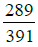when reduced to the lowest terms is
(a)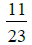(b)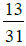(c)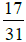(d)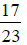Ans.
(d) Because HCF of 289 and 391 is 17 and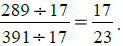Q.16. The greatest number which divides 134 and 167 leaving 2 as remainder in each case is
(a) 14
(b) 17
(c) 19
(d) 11

Ans.
(d) Because dividing 134 and 167 by 11, remainder is 2 in each case.

Q.17. The LCM of 24, 36, 40 is
(a) 4
(b) 90
(c) 360
(d) 720
Ans. (c) Because 360 is the least multiple of 24, 36 and 40.

Q.18. The LCM of 12, 15, 20, 27 is
(a) 270
(b) 360
(c) 480
(d) 540
Ans. (d) Because 540 is the least multiple of 12, 15, 20 and 27.

Q.19. The smallest number which when diminished by 3 is divisible by 28, 36 and

45, is
(a) 1257
(b) 1260
(c) 1263
(d) none of these
Ans. (c) Because 1263 – 3 = 1260 is divisible by 28, 36 and 45.

Q.20. The HCF of two co-primes is
(a) the smaller number
(b) the larger number
(c) 1
(d) none of these
Ans. (c) Because HCF of two co-prime number is always 1.

Q.21. If a and b are co-primes, then their LCM is
(a) 1
(b)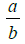(c) ab
(d) none of these
Ans.
(c) Because HCF of a and b, two co-primes is 1.
∴ LCM = a × b = ab.

Q.22.The product of two numbers is 2160 and their HCF is 12. The LCM of these numbers is
(a) 12
(b) 25920
(c) 180
(d) none of these
Ans.
(c) Because LCM of two numbers = Product of these number/their HCF i.e. 2160/12 =
180.

Q.23. The HCF of two numbers is 145 and their LCM is 2175. If one of the numbers is 725, the other number is
(a) 290
(b) 435
(c) 5
(d) none of these
Ans.
(b) Because second number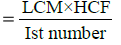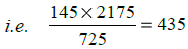Q.24. The least number divisible by each of the numbers 15, 20, 24, 32 and 36 is
(a) 1660
(b) 2880
(c) 1440
(d) none of these
Ans.
(c) Because LCM of 15, 20, 24, 32 and 36 = 1440.

Q.25. Three bells toll together at intervals of 9, 12, 15 minutes. If they start tolling together, after what time will they next toll together ?
(a) 1 hour
(b)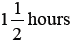(c)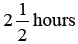(d) 3 hours
Ans.
(d) Because LCM of 9, 12, 15 is 180.
∴ 180 minutes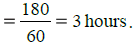Offer running on EduRev: Apply code STAYHOME200 to get INR 200 off on our premium plan EduRev Infinity!

## Mathematics (Maths) Class 6

191 videos|224 docs|43 tests

,

,

,

,

,

,

,

,

,

,

,

,

,

,

,

,

,

,

,

,

,

;